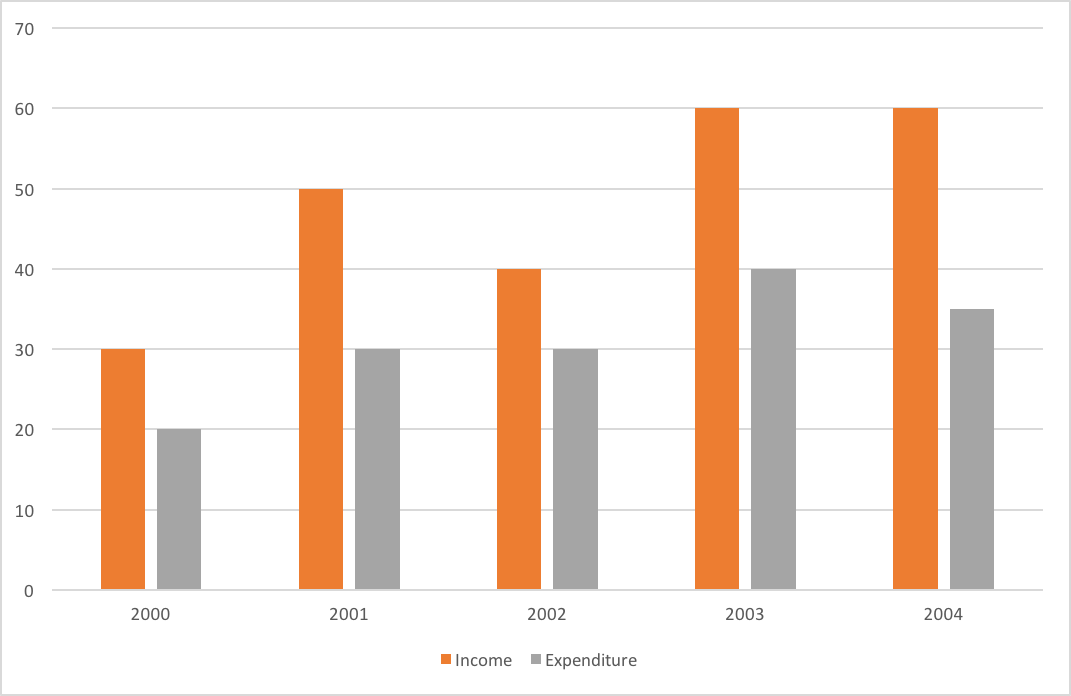## SSC CGL Quant Test 17

Instructions

The graph shows Income and Expenditure(Rs. in lakhs) of a company.Study the graph and answer the questions.Question 1

The expenditure from 2002 to 2003 increased by

Question 2

The income in 2002 was equal to the expenditure in the year

Question 3

The profit was maximum in the year

Question 4

The difference in profit between 2001 and 2002 is

Question 5

The number of years in which the income exceeds the average income is